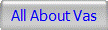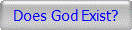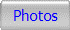Proof of the Existence of God The Ingenious Numeration of Three Constants

Expanded Highlights of the Evidence Illustrated in Document Alpha

Vasilios Gardiakos

Throughout the many centuries pi (π) has been examined and dissected in countless ways. The fascination with pi continues to the present. To this day no one has noticed anything unusual about pi.Only one entity could manipulate these three constants to produce oddities that we can detect. Only one entity could do this specifically on these three constants and not on other numbers. It seems that God not only could but has formed the geometry of space and even defined the fundamentals of mathematics. He ingeniously manipulated the geometric fabric and the default mathematics of the universe so that we can detect and analyze the oddities and conclude that only a great intelligence can accomplish this and therefore we can know that God exists.

Note: Document A may look complicated but it is not! It is fairly simple to understand. Any one with a little knowledge of math can understand it. The many arrows on Document Alpha though they may look confusing or distracting are there to point or reference the numbers. The highlights below are discussed step by step in detail to explain the oddities. Once you understand Document Alpha you will realize its significance.

1) Pi (pi = 3.141592653...) starts with 3_4_5 which is the smallest Pythagorean Triplet possible forming the Pythagorean triangle. This triplet, 3_4_5 can be recognized as the representation of the Pythagorean Theorem. Also in pi the sequence 1_ _2_ _3. In √2 early the sequence1_2_3  and 4_ _3_ _2.

2) In pi, 31, 41 and 59 are primes (3.1 41 59...). No formula has been discovered that will produce all the prime numbers however 41 + n squared + n, 59 + 4n squared + 4n and 31 + 2n squared + 4n are three most productive formulas known to yield prime numbers. Both 41, 59 and 31 are used. See 5) bellow. Right at the start of pi there are three very productive prime number generators, 31, 41 and 59. What are the odds of this happening by chance?

The great mathematician's Leonhard Euler's formula 41 + n squared + n turns out to be surprisingly good for large values of n. A computer showed that for the primes under ten million, the formula generated primes 47.5 percent of the time. The formula works even better for lower values of n. For values of n under 2,398, there's' an even chance of getting a prime. And for values under 100 the formula yields 86 primes and only 14 composite or non-prime numbers.

For n=0, the formula yields the prime number 41. For n=2, the prime number 47. Indeed, as n takes on successive values from 0 to 39, Euler's formula yields nothing but primes.

Stanislaw Ulam, a well known and respected mathematician and his coworkers discovered another prime generating formula that is almost as good as Euler's. The formula 59 + 4 n squared + 4n has a success rate of 43.7 percent.

Steve Homewood a determined and ingenious mathematician based in the U.K. announce on March 7, 2009 that he had solved the A31 problem. He discovered the formula 31 + 2n squared + 4n generates prime numbers 43.5% of the time for all answers up to 10,000,000. So as he says “So, no, 31 has not been overlooked”.

3) In pi, 31, 41 and 59 oddly their prime order, being the 11th, 13th and 17th primes are themselves primes. More oddly 11, 13 and 17 are consecutive primes. Working with 11, 13 and 17, oddly 1+1+1+3+1+7=14 and 11+13+17= 41 (3.14159...). One more oddity, in 11, 13, and 17, 1+1=2, 1+3=4 and 1+7=8 a 2-4-8 progression. Also 31+ 41+ 59=131 and 3+1+4+1+5+9= 23, both sums are prime numbers.

4) Pi starts with the prime number 31 which is also a Mersenne Prime. The cube root of 31=3.141380652 which is within .00675% of pi. The first 8 digits of pi 3+1+4+1+5+9+2+6=31 also 1+4+1+5+9+2+6+3=31. There is no zero in the first 31 digits of pi. Note, 1+2+4+8+16=31 and (from 16=31) 16x31=496. The third perfect number is 496.

5) Odd that pi starts with 314159 and its mirror image 951413 are both prime numbers. About 1 in 7 primes is the reversal also a prime.

These are the four known pi forward primes:
3, 31, 314159 and 31415926535897932384626433832795028841
So in addition to 314159 being a reversible prime it is also a rare pi forward prime.

A lesser oddity, the first even number in pi, 314 divided by two equals 157 which is a prime. Also 14, an even number divided by two equals 7, a prime.

6) Pi = 3.14159265358979323... It is very odd that a group of eight small different contiguous primes: 3, 14159, 2, 653, 5, 89, 7, 9323 are right at the start of pi. Many and possibly infinite small (numbers with five or fewer digits) and large (greater than five digits) different contiguous primes may exist after 9323. As it turns out right after the 9323 prime the next contiguous prime is: 846264338327........303906979207, it is 3057 digits long. So if pi started with 8462... the first prime would be 3057 digits long. See: http://www.primepuzzles.net/problems/prob_018.htm

It will be interesting to see how many digits the average contiguous prime has. Perhaps more interesting may be to find how scarce are groups consisting of eight small contiguous primes of which none of the prime numbers are duplicated.

7) Starting with the first digits in √2, 1_1_2_3; the first digits in S, 8_6_2_9 and the first digits in pi, 2_5_4_3 (3_4_5_2 inverted) are prime numbers.

8) Pi and √2 have the 2653, 3562 cross-mirroring and the 141,414 cross-symmetry at DEF/2. (see alphanumeric locators on the sides of Document A) The mathematical block at E/3 reveals several vertical and diagonal oddities. The repeating "88" ties pi and √2 to S.

9) In √2 (√2= 1 .4 1 4 2 1 3 5 6 2 ...) early 1+_2+_3=_6. Not only does it equal 6, a perfect number but this is an example of how to derive the first perfect number which is 6. Again in √2 early14+14=28, 28 being the second perfect number. Furthermore sum of the digits 1+4+1+4 = 2+8 = 10 which is the fourth triangular number

10) √2 starts with the Fibonacci sequence 1_1_2_3 in which 11, 23 and 1123 are primes.
Also note:
√2 = 1 .4 1 4 2 1 3 5 6 2 3 7 3...
Fibonacci sequence: 1, 1, 2, 3, 5, 8, 13...
In
√2 the Fibonacci sequence is included: 1, 1, 2, 3, 5, 8(6+2), 13(3+7+3). Very odd that seven numbers of the Fibonacci sequence are found at the start of the square root of 2.

11) The 1_1_2 in √2 is in an identical position right at the beginning as the 3_4_5 in pi. Both 3,4,5 and 1,1,√2 can be represented by a triangle. See Document A.

12) √2 has a strong 7 occurrence. Many consecutive numbers add up to multiples of  7. In √2 early 14-21-35 are multiples of 7. Note that 22/7 equals pi within 0.04025%. See G/1-9 on Document A.

13) S (Side=) starts with 88. Numbers 88, 8 and 8 are prominent in 62(6+2=8) and 26(2+6=8) add up to 88 and are in a sense self-referential. At DF/12 Pi and √2 also refer to 88: 62+26=88, the 62 from √2 and the 26 from pi oddly 6226 is found in S. Pi and √2 refer to 88: 35+53=88. See more 88 oddities on Document A.

14) The 22 oddity: 11, 22 and 88 figure prominently in pi, √2 and S. Also 88 is present in some way in all three constants and is a multiple of 11 and 22.

15) S=.886226925
886  22  6925
8+8+6=22=6+9+2+5
Also interesting: 88+6=94=69+25 also 69-25=44=2x22

16) S=.886226925, so that 886, 862, 622, 226, 692 (odd numbers are ignored):

abc-cba=d,      d/x=22
886-688=198, 198/9=22
862-268=594, 594/27=22
622-226=396, 396/18=22
226-622=-396, -396/18=-22
692-296=396, 396/18=22

abcdef,   abcdef/x=22
886226, 886226/40283=22
fedcba,   fedcba/x=22
622688, 622688/28304=22

263538, 263538/11979=22

17) The initial consecutive digits in pi, √2 and S can add up to 41 (pi=3.141...):
pi: 1+4+1+5+9+2+6+5+3+5 = 41
√2: 4+1+4+2+1+3+5+6+2+3+7+3 = 41
S: 8+8+6+2+2+6+9 = 41
Multiply 41 by 3 (the three constants) which is equal to 123. Now 1+2+3=6 which is a perfect number.

18) Very strange the E-5 mathematical block is the result of the "1415" or 14-15 in Pi and "4142" or 41-42 at identical place relative to the decimal point on √2. This block contains several vertical and diagonal and prime number oddities.

===========================
Conclusion

The "oddities" explained above is
the evidence that proves that God
intentionally numerated the three
constants so that we can become
aware that He exists.

Check out the criticism: Page 1  Page 2  Page 3  To Page 4

===========================

And Now For The Fun Stuff

On a Humorous Note (S=886226925):

It takes 62 muscles to frown
and only 26 to smile.
(I am pushing things a bit here)Photo V. Gardiakos - My 1973 trip to New Orleans

On a Telephone Note:

The telephone area code of St Louis
home of The Gateway Arch is 314
and has a zip code of 63141
(I am pushing things a lot here)

On a Musical Note (S=886226925):

88 represents the 88 keys of a piano.
(now this is ridiculous connection so I
include it only to extract a smile from you.

Notes:

A. All of the underlined hyperlinks in this page are not in the typical blue but are in the color of this font.

B. "_" The under score represents a digit in the sequence that is not shown. Sometimes I underscore a digit for emphasis. The underscore under a number does not indicate that it is a hyperlink.

C. Pi (π) is the ratio of the circumference of a circle to its diameter. Pi is the most well known non-integral mathematical constant. Pi is equal to 3.141592653... which is infinitely long. Pi shows up in many equations where you don't expect it. It is the 16th letter of the Greek alphabet.

D. 2 = 1.414213562373... ("√2" is the mathematical representation of square root of two)

E. S=0.886226925... which is 1/2 times the square root of pi. It is gamma(3/2), and is sometimes also called (1/2)! the factorial of 1/2. Herein "S" is used for "side" for the sake of convenience.

F. Mersenne Primes: 2, 3, 5, 7, 13, 17, 19, 31, 67, 127, 257 ... They become more rare as the value increases.

G. 22/7=3.142857... = pi within 0.04025%. The first theoretical calculation of a value of pi was that of Archimedes of Syracuse (287-212 BCE), one of the most brilliant mathematicians of all time. Archimedes worked out that 223/71 < π < 22/7. Interestingly 22/7 is also a  member of the continued-fraction series for pi. Continued fraction approximations can be used to get "optimal" rational approximations for any number. By "optimal" we mean the closest approximation that is possible with a given-size numerator and denominator. The continued fraction approximations for π are: 3/1, 22/7, 333/106, 355/113, 103993/33102, 104348/33215, 208341/66317, 312689/99532, 833719/265381, 1146408/364913, ...

H. 7 is the smallest number whose reciprocal has a pattern of more than one repeating digits: 1/7 = 0.142857142857... It is also the smallest number for which the digit sequence is of length N-1 (the longest such a sequence can be). The next such numbers are 17, 19, 23, 29, 47, 59, 61, 97, 109, 113, ... (Sloane's integer sequence A006883)

I. 7 is considered "lucky" by many people and given much spiritual significance. The early religious and cultural use of the 7-day week almost certainly arose from the fact that the moon goes through its 4 phases in a bit over 28 days, which divides nicely into 7 days per phase. These man made numeral references may be part of numerology however they are excluded as being significant or relevant in the oddities of Document A.

J. What is a prime number?

A prime number is a positive integer that has exactly two positive integer factors, 1 and itself. For example, if we list the factors of 28, we have 1, 2, 4, 7, 14, and 28. That's six factors. If we list the factors of 29, we only have 1 and 29. That's 2. So we say that 29 is a prime number, but 28 isn't. Another way of saying this is that a prime number is a whole number that is not the product of two smaller numbers.

Note that the definition of a prime number doesn't allow 1 to be a prime number: 1 only has one factor, namely 1. Prime numbers have exactly two factors, not "at most two" or anything like that. When a number has more than two factors it is called a composite number.

Here are the first few prime numbers: 2, 3, 5, 7, 11, 13, 17, 19, 23, 29, 31, 37, 41, 43, 47, 53, 59, 61, 67, 71, 73, 79, 83, 89, 97, 101, 103, 107, 109, 113, 127, 131, 137, 139, 149, 151, 157, 163, 167, 173, 179, 181, 191, 193, 197, 199, etc.

Prime numbers sequences are useful as they do not occur in nature. Only intelligent beings can create a prime number sequence. In the search for extra terrestrial intelligence any detection of a  prime number sequence will be recognized as being created by a intelligent beings.

Mirror prime or reversible prime number or emirp (prime spelled backwards) is a prime that gives you a different prime when its digits are reversed.

K. A31

Prior to March 6, 2009 only Euler's (1707 - 1783) 41 + n squared + n and Ulam's (1909 - 1984) 59 + 4n squared + 4n simple polynomial formulas were known to be very productive in generating prime numbers. Since pi starts with 3.14159... and if God intentionally numerated pi with 41 and 59 as prime number generators then it is logical that 31 could also be used to do likewise.

Steve Homewood a very talented mathematician based in the U.K. took up the painstaking task to solve the A31 problem and March 6, 2009 announce that he had done so! Referring to my question "Has 31 been overlooked in the search for other productive numbers?" Homewood replied with “So, no, 31 has not been overlooked”. He discovered the formula 31 + 2n squared + 4n generates prime numbers 43.5% of the time for all answers up to 10,000,000. There are other simple polynomial prime number generators but are not as productive as these three.

Nothing to do with A31 and thus not significant but nevertheless interesting oddity: 31, 331, 3331, 33331, 333331, 3333331, and 33333331 are all prime numbers.  But the pattern is broken with the next number in this sequence: 333333331 is not prime. Back to 2)

L. What is the Fibonacci Sequence?

Fibonacci Sequence:1, 1, 2, 3, 5, 8, 13, 21, 34 ... The Fibonacci series is formed by starting with 0 and 1 and then adding the latest two numbers to get the next one. Each number is the sum of the previous two. Surprisingly, Fibonacci numbers can be used to calculate pi.

M. What is a perfect number?

A perfect number is a number that it is equal to the sum of its own divisors (other than itself). Examples:
6 = 1 + 2 + 3
28 = 1 + 2  + 4 + 7 + 14

N. What is a triangular number?

A triangular number or Tetraktys is the number of dots that may be arranged in an equilateral triangle: 1, 3, 6, 10, 15, 21, 28, 36... In general n(n + 1)/2.

O. What is the definition of "oddity" when referring to Document A?

Oddity defined: abnormal unusual strange atypical extraordinary surprising amazing weird uncommon unexpected unusual unpredicted unforeseen remarkable notable noteworthy.

In Document A "oddities" refers to simple unexpected occurrences that include prime numbers, Fibonacci sequence, perfect numbers, Pythagorean triplet etc. One could write countless unique equations to fit every irrational or random number and call it an oddity. This force to fit technique is not used in Document A. It is important to find simple oddities as there are limits to simplicity like counting, adding, subtracting and multiplying. Complexity has not limits. I suspect it is more difficult to numerate simple oddities as opposed to complex ones as complexity allows you an unlimited course of action. All the oddities found in Document A are of the simple type. No complex equations, complex algorithms or complex formulas are used to find the oddities. Perhaps natural  rather than contrived may be a way to describe the 19 or so oddities in the webpage.

Another reason simple oddities are more relevant is because they are easier to be spotted and recognized as such. If the oddities are intentional then they should be easy to spot or what would be the sense of numerating them in the first place?

One oddity may be noteworthy but additional oddities make it significant. The more oddities the more noteworthy and significant it is. The 19 or more oddities in Document A is enough evidence to conclude that a powerful intelligence namely God numerated pi.

By the way one equation that many mathematicians would agree is an oddity is e^(i*pi)=1. To further clarify, I use "oddity" with a similar meaning.

Comment:
God herein means the Pythagorean God and not any of the Gods of the various religions.______________________________________________________